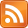[This post is likely only to be of interest to you if you've read the paper under discussion.]

The paper Risks from Learned Optimization in Advanced Machine Learning Systems is written in natural language. It discusses the possibility of two functions — the objective function of the base optimizer, and the objective function of the mesa optimizer — being equal or unequal. As Samuel Marks pointed out during a presentation, in this informal setting, it's not clear that these functions even have the same type. Here's two formalizations where it's clearer what we mean when we discuss equality between these two functions.

(These ideas were birthed at a whiteboard discussion with Samuel Marks, Buck Shlegeris, Ben Weinstein-Raun, Scott Garrabrant and myself. Any errors are mine.)

The issue is that the objective function of the base optimizer is evaluating models, but the objective function of the inner optimizer is probably evaluating something else. We can smooth out the types by changing everything into Turing machines.

We're going to run an algorithm to optimize some function on Turing machines, considered as strings over $\text{TM} = \{0, 1\}$. We have a function $U : \text{TM} \rightarrow \mathbb{R}$ which scores our machines on this metric.

Example: in the case of evolution, $U$ is: how well does this machine self-replicate?

Suppose we have $m \in \text{TM}$ such that $m$ is a mesa-optimizer. What $m$ does when run is simulate some ten other Turing machines, evaluate which is the best one according to some internally represented function $U_m : \text{TM} \rightarrow \mathbb{R}$, and act like that one.

Example: in the case of evolution of humans, $m$ is a human brain and $U_m$ is "does behaving like this seem like it would suit my goals?"

• Note that $U_m$ is not a utility function that takes in states of the world and spits out utilities. Its domain is Turing machines.
• Note that the domain of $U_m$ may be only a subset of the domain of $U$; that is, $U_m$ may be a partial function from $\text{TM} \rightarrow [0, 1]$. A human does not need to be able to evaluate all possible minds. It just needs to be able to evaluate the minds it could act like.

Now we have $U$ and $U_m$ of the same type, so we can meaningfully say that inner alignment is when $U = U_m$ (at least, on the domain of $U_m$).